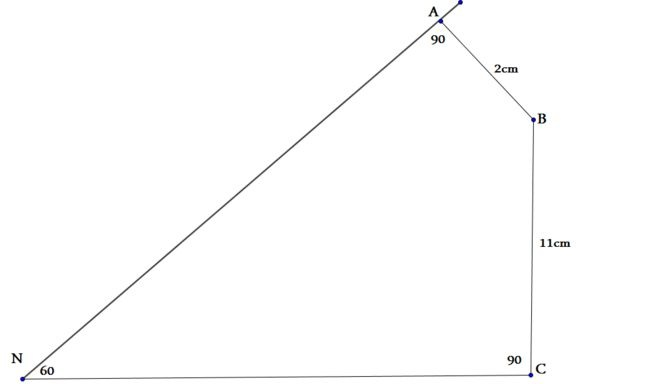# The bouncy problem

Geometry Level 3Given $\angle BAN$ and $\angle BCN$ are right angles and that $\angle ANC = 60^\circ$. Find the length of straight line $BN$.

×

Problem Loading...

Note Loading...

Set Loading...